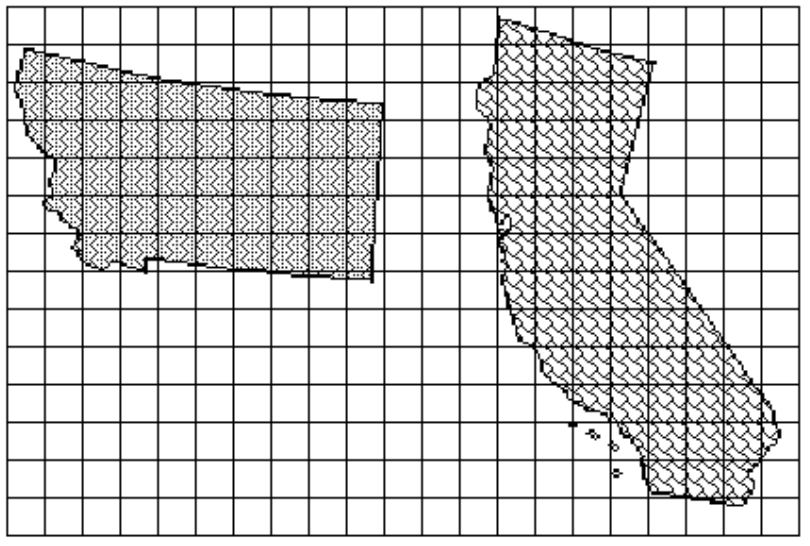### Home > ACC7 > Chapter 1 Unit 1 > Lesson CC3: 1.1.4 > Problem1-39

1-39.

Estimate the areas of Montana and California using the grid below. Which state has the greatest area? Compare the area of Montana to the area of California. Explain how you estimated the area of each state. Homework Help ✎Find the number of whole squares each state contains.

Find the number of partial squares each state contains.

Assume that the partial squares have an average area of half a square. Use this assumption to estimate the area of each state.